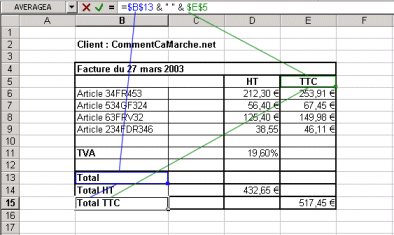## Concatenation Operator

Spreadsheets generally use an oeprator, called a concatenation operator , that allows two text values to be added together. The concatenation operator, written as &, is used as follows:In the above example, the cell value B15 ("Total TTC") is automatically calculated by concatenating the value of cell B13 (whose absolute reference is written \$B\$13), a chain made of a space " ", and the value of cell E5 (whose absolute reference is written \$E\$5), thanks to the following formula:

`=\$B\$13 & " " & \$E\$5`